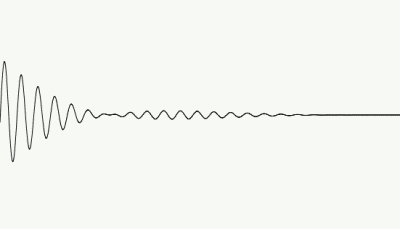# Lecture 3: Nature of Light

Textbook: Chapter 5

## Properties of Waves

### Wave is periodic disturbance in space that oscillates

• The distance between wavecrests is called the wavelength. Wavelength is denoted with the Greek letter "lambda" &lambda
• The frequency "f" is the number of times that the wave oscillates in a second.
• Time it takes for one complete oscillation is called period "T"
T = 2 &pi / f
• Most fundamental case: The wave travels. Position of crests move at velocity V . Velocity depends on properties of the medium.
• For any type of wave, the frequency, wavelength and wave speed are related by
f &lambda = V
A wave with long wavelength vibrates slowly (frequency is small) and a short wavelength wave vibrates quickly.

### Frequency of the wave determines its energy. Travelling wave transports energy.

• When velocity of a wave changes, its frequency remain unchanged, but wavelength changes — f=V/&lambda =const. Why ? Energy conservation !
(animations courtesy of David Harrison collection at UofToronto)

Next

## Light is an electromagnetic (EM) wave

### An Electromagnetic Wave is an alternating oscillations of the Electric (E) and Magnetic (B) fields

• The EM wave travels in vacuum at a constant speed
V = c = 3 x 108m/s
• f &lambda = c
• If the EM wave has wavelenth in the visibile range
400 nm < &lambda < 700 nm
our eyes can detect the EM wave. nm = nanometer = 10-9m)
• Our eyes interpret EM waves with different wavelengths in the visible range as different colours.
• In order of long wavelength to short wavelength:
red, orange, yellow, green, blue, indigo, violet (ROYGBIV)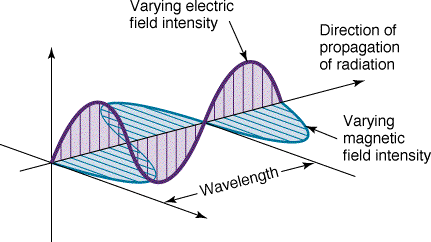### EM Waves are produced my moving electric charges

Animation is here or here (courtesy of David Harrison at UofToronto)

Back Next

### But visible light is not the only type of EM wave!

Order of types of EM waves from long wavelength to short wavelength: (also order of small frequency to high frequency)
 Type Wavelength Range Radio wavelength > 1 cm Microwave 1 mm < wavelength < 1 cm Infrared 700 nm < wavelength < 1 mm Visible 400 nm < wavelength < 700 nm Ultraviolet 20 nm < wavelength < 400 nm X-rays 0.1 nm < wavelength < 20 nm Gamma rays wavelength < 0.1 nm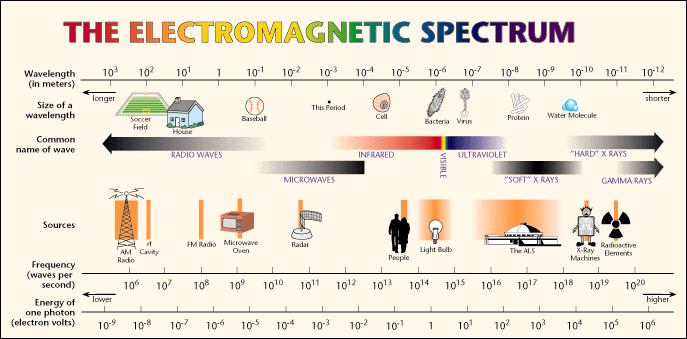Richard Feyman on the EM waves and light

Back Next

## Light Rays versus Light Waves

### Far from the source the wave is planar

the crests of the wave form planes (in 3D) or straight lines (in 2D)
• Planar wave has well-defined direction of propagation, thus we can think about light rays
Physicists say "we can use geometrical optics approximation here"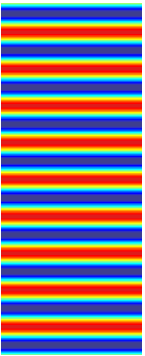### Point source emits spherical waves.

• Far from the source, segment of spherical surface looks like plane and we speak about rays . EM waves from distant stars is very planar !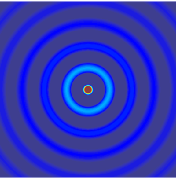Diffraction

Back Next

## Particle Properties of Light

 Light also behaves as though it comes in particles called photons. A photon has a wavelength and frequency associated with it. The Energy carried by the photon is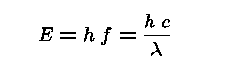In this equation, h is Planck's constant: h = 6.625 x 10-34J s. Since fblue > fred, blue photons carry more energy than red photons! (Opposite from most people's intuition)